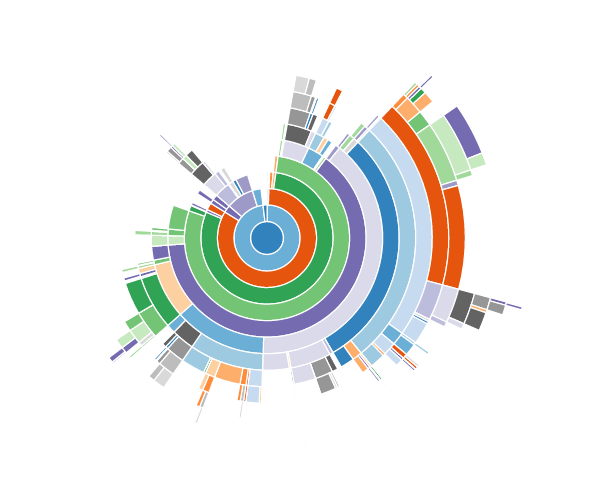# Performance of Pandas Series vs NumPy Arrays

I recently spent a day working on the performance of a Python function and learned a bit about Pandas and NumPy array indexing. The function is iterative, looping over data and updating some row weights until it meets convergence criteria. I tried to do as much processing as I could before the loops, but some indexing (and of course arithmetic) had to stay inside the loops.

When I looked at profiles of the function almost all of the time was being spent doing indexing on Pandas Series objects. A quick investigation shows that indexing Series objects is quite slow compared to NumPy arrays. First, some setup:

``````In : import numpy as np

In : import pandas as pd

In : np.version.version
Out: '1.8.2'

In : pd.version.version
Out: '0.14.1'

In : a = np.arange(100)

In : aa = np.arange(100, 200)

In : s = pd.Series(a)

In : ss = pd.Series(aa)

In : i = np.random.choice(a, size=10)
``````

And here’s the performance comparison:

``````In : %timeit a[i]
1000000 loops, best of 3: 998 ns per loop

In : %timeit s[i]
10000 loops, best of 3: 168 µs per loop
``````

Indexing the array is over 100 times faster than indexing the Series. This shows up in arithmetic too, because Pandas aligns Series on their indexes before doing operations:

``````In : %timeit a * aa
1000000 loops, best of 3: 1.21 µs per loop

In : %timeit s * ss
10000 loops, best of 3: 88.5 µs per loop
``````

If the Series are already aligned that is wasted processing. (You can also see this as an IPython Notebook.)

Why is Pandas so much slower than NumPy? The short answer is that Pandas is doing a lot of stuff when you index into a Series, and it’s doing that stuff in Python. As an illustration, here’s a visualization made by profiling `s[i]`:Each colored arc is a different function call in Python. There are about 100 calls there.

By contrast, here’s the visualization made by profiling `a[i]`:There’s actually nothing to see because array indexing goes straight into the NumPy C extensions, and the Python profiler can’t see what’s going on there. (The visualizations were made with SnakeViz.)

With this in mind I rewrote my function (and its supporting code) so that during the loop all the data would be in plain NumPy arrays. The inputs are DataFrames and Series, which I reorganize into arrays and scalars. At the end I transform the array of weights into a Series with the appropriate index. Here are the before and after of the module I was working on, as well as the diff. (The `household_weights` function is the high level entry point.)

I should note that using Pandas is fast enough most of the time, and you get the benefit of Pandas’ sophisticated indexing features. It’s only in loops that the microseconds start to add up to minutes.

## 4 thoughts on “Performance of Pandas Series vs NumPy Arrays”

1.somada141 says:

Very interesting post! Seems that all the fancy Pandas functionality comes at a significant price (guess it makes sense since Pandas accounts for N/A entries and heterogeneous arrays). Nonetheless, NumPy itself burns quite a bit of time checking index bounds etc so if you want to supercharge things you’re gonna have to go to Cython :)

2.ilan says:

interesting post.
you can use panda and the nice thing that it brings

when you need performance, you can do :

d = s.values
%timeit d[i]
1000000 loops, best of 3: 998 ns per loop

and you will get the same performance , as panda use numpy eventually

1.jiffyclub says:

That is essentially what I’m doing.

This site uses Akismet to reduce spam. Learn how your comment data is processed.